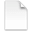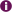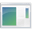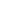Share

# .gcw

File TypeMicrosoft Mathematics Worksheet
File Format Proprietary (Microsoft)
Usage Open manually
Popularity ★ ★ ★ ★ ☆  Common
Description

A GCW is a worksheet created with Microsoft Mathematics (formerly Microsoft Math). It may contain formulas, graphs, and handwritten equations. By saving a GCW file, a user can save his or her work on a specific math or science problem, then resume the work at a later time.About Microsoft Mathematics

Microsoft Mathematics is an educational tool designed for visualizing mathematical concepts. It includes a graphing calculator, a step-by-step equation solver, a unit conversion tool, and a library of formulas and equations. Mathematics supports both direct input and hand-written equations.

Open With
 WindowsMicrosoft Mathematics
Published June 7, 2011
Information from the PC File Extensions Reference
https://pc.net/extensions/gcw